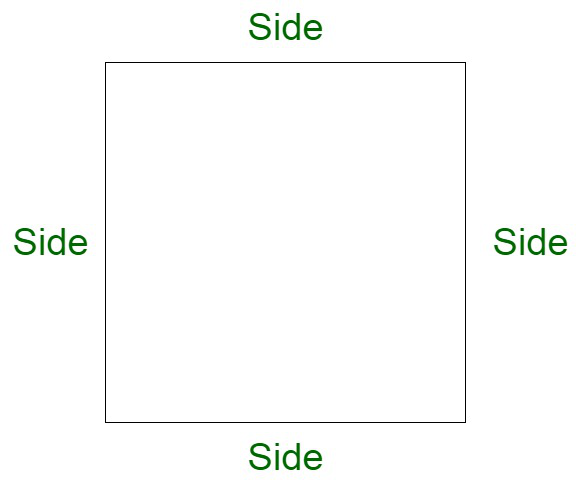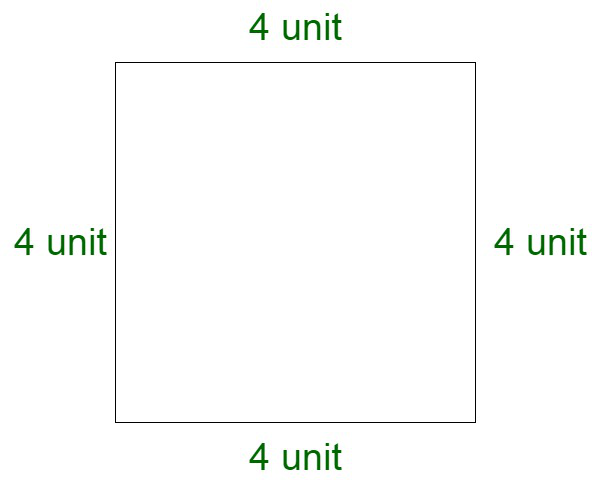Open in App
Not now

# If the perimeter and area of a square are equal, calculate the side of the square.

• Last Updated : 21 Nov, 2021

Square is a closed two-dimensional figure with four sides and four corners. The length of all four sides is equal and parallel to each other.

A square is a quadrilateral in which:

• The opposite sides are parallel.
• All four sides are equal.
• All angles measure 90°.

### Perimeter of the square

The length of the boundary of a square can be calculated by performing the summation of all its sides, which is given by,

Perimeter of a square = side + side + side + side.

Therefore, Perimeter of Square = (4 × Side) units### Area of square

The area of a square is the space enclosed within its boundary. It is given by the product of any of its two sides and denoted in sq. units. By formula, we have,

Area of a square = Side × Side

Let us assume the side of the square to be equal to S.

Therefore,

Area =  S2

### If the perimeter and area of a square are equal, calculate the side of the square.

Solution:

Since, we know,

⇒ Perimeter of a square

Perimeter of a square = Side + Side + Side + Side

Perimeter of a square = 4 × Side  ……….(1)

⇒ Area of a square

Area of a square = Side × Side

Area of a square = (Side)2  ……….(2)

Let us assume the side of the square to be ‘s’.

Further, if we equate (1) and (2) as per the problem

We will get;

Perimeter of a square = Area of a square …..(given)

⇒ 4 × Side = Side × Side

⇒ 4 × s = s × s

⇒ 4 × s = s2

Taking L.H.S ‘s’ to R.H.S

⇒ 4 =⇒ 4 = s

⇒ s = 4

Side = 4 unit

Therefore,

If the side of the square is 4 units, then its perimeter and area will be equal

On checking square with 4 units side will give equal perimeter and area.

⇒ Perimeter of a square = 4 × Side

Perimeter of a square = 4 × 4

Perimeter of a square = 16 unit

⇒ Area of square = Side × Side

Area of square = 4 × 4

Area of square = 16 unit2

Hence,

We can see that if the side of the square is 4 units, then the perimeter and area of the square are equal.### Sample Questions

Question 1. Find the perimeter and area of a square with a side of 14 m?

Solution:

Here we have to find the perimeter and area of a square

As we know that

⇒ Perimeter of a square = 4 × Side

Perimeter of a square = 4 × 14

Perimeter of a square = 56 m

⇒ Area of square = Side × Side

Area of square = 14 × 14

Area of square = 196 m2

Therefore,

The perimeter of a square with a side of 14 m is 56 m

The area of a square with a side of 14 m is 196 m2

Question 2. Assume that if the perimeter of a square ground is 80 m. Then find the area of the square ground?

Solution:

Here we have to find the area of the square ground

Given:

Perimeter of the square ground = 80 m

As we know that

Perimeter of a square = 4 × side

Perimeter of a square = 4 × s

80 = 4 × s

s =s = 20 m

Side of the square ground is 20 m.

Now,

Area of a square = Side × Side

Area of a square = s × s

Area of a square = 20 × 20

Area of a square = 400 m2

Therefore,

The area of the square with a perimeter of 80 m is 400 m2

Question 3. Find the perimeter of the square if the area of the square is 121 cm2

Solution:

Here we have to find the perimeter of the square

Given:

Area of the square = 121 cm2

As we know that

Area of the square = Side × Side

Area of the square = s × s

Area of the square = s2

121 = s2

s =s = 11 cm

Side of the square = 11 cm

Further,

Perimeter of the square = 4 × side

Perimeter of the square = 4 × 11

Perimeter of the square = 44 cm

Therefore,

Perimeter of the square with an area of 121 cm2 is 44 cm.

Question 4. If the perimeter of a square floor is 88 m, then find the cost of carpeting the floor at the rate of ₹20 per meter?

Solution:

Here we need to find the cost of carpeting the floor

Given:

Perimeter of the floor = 88 m

As we know that

Perimeter of the square = 4 × side

Perimeter of the square floor = 4 × s

88 m = 4 × s

s =s = 22 m

The side of the square is 22 m

Further,

Carpet required = Area of the floor

Area of the square floor = s × s

Area of the square floor = 22 × 22

Area of the square floor = 484 m2

Now,

Cost of carpeting = Area of the square floor × ₹20

Cost of carpeting = 484 × 20

Cost of carpeting = ₹9680

Therefore,

The cost of carpeting the square floor is ₹9680.

Question 5. Calculate the perimeter and area of a square box with a side of 44 cm?

Solution:

Here we have to find the perimeter and area of a square box

As we know that

⇒ Perimeter of a square = 4 × Side

Perimeter of a square box = 4 × 44

Perimeter of a square box = 176 cm

⇒ Area of square = Side × Side

Area of square box = 44 × 44

Area of square = 1936 cm2

Therefore,

The perimeter of a square with a side of 44 m is 176 cm

The area of a square with a side of 44 m is 1993 cm2.

My Personal Notes arrow_drop_up